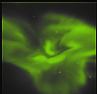Next: Pixel field-of-view Up: The Forward model - Previous: The Forward model -   Contents

# Voxel -- Pixel field-of-view intersection

In order to calculate the contribution from a voxel to a pixel in an image, the volume of the intersection between the pixel field-of-view and the voxel has to be calculated. The intersection between a voxel and the pixel field-of-view is a body with between four and ten faces. In order to avoid having to calculate the volume of the complex body with all its bounding planes, the intersection volumeis approximated by the area spanned by the pixel field-of-view,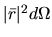, at distance,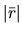, to the voxel multiplied by the pixel line-of-sight intersection length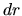:(5.3)

In order to study the error in the approximation, we turn to the corresponding two-dimensional example illustrated in figure 5.2. The manageable problem is a narrow circle sector intersecting three voxels with intensities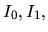and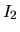, respectively. For a voxel representation of a function in three dimensions to model the function well the function should have slow spatial variations compared to the size of the voxels, i.e. neighbouring voxels should have intensities that do not differ much: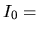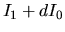(5.4)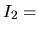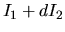(5.5)

Where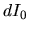and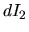are small compared to.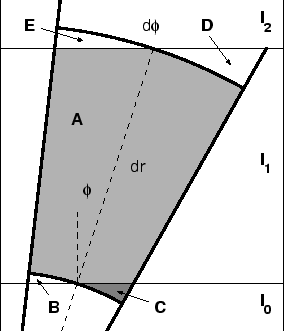The true contribution from voxel 1 is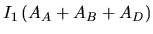. The proposed approximation is that the contribution from voxel 1 is given by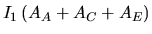. Hereis the area of region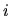. In order to calculate the difference between the exact and the approximate contributions, we calculate the true and approximate contributions from the region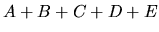: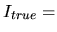(5.6)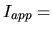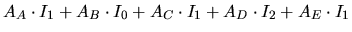(5.7)

Calculating the difference between the true and the approximate contribution, and using equations (5.4 and 5.5), gives: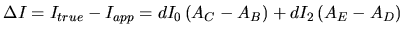(5.8)

After doing some geometry and some algebra the areas of the different regions can be obtained: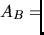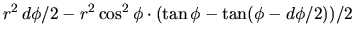(5.9)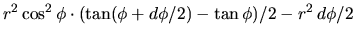(5.10)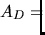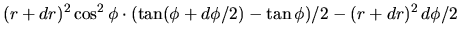(5.11)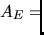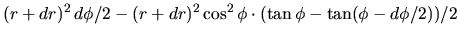(5.12)

Substituting these equations into equation (5.8) and expanding to the third order in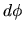, gives:(5.13)

Here it is seen that the approximate contribution,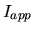, is correct to within the third order inand the second order in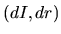. With values typical for ALIS and auroral tomography this gives relative errors that are of the order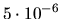.

As proven the approximation for voxel-pixel intersection is good enough. A significantly more interesting topic is whether or not the voxel approximation of the continuous volume emission distribution is good enough. As a first test a simple model with only 1 voxel in altitude and a 2-D Gaussian intensity distribution is used with various sizes of the voxels. These voxel representations are projected down onto images with 256 by 256 pixels as shown in figure 5.3.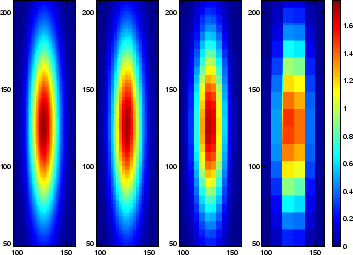It can be seen that for voxel sizes that are comparable to the typical dimensions of the structures in the distribution the images become coarse. Looking at the differences between images projected from voxels with different resolutions, as presented in figure 5.4,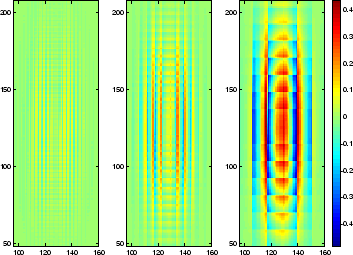it is seen that the voxel representation needs to be a posteriori justified, or that the number of voxels must be increased when the result of the tomographic inversion shows structures in the volume emission distribution which have widths of only a few voxels.Next: Pixel field-of-view Up: The Forward model - Previous: The Forward model -   Contents

copyright Björn Gustavsson 2000-10-24